Area Of 2 D Shapes - Definition with Examples

The Complete K-5 Math Learning Program Built for Your Child

• 30 Million Kids

Loved by kids and parent worldwide

• 50,000 Schools

Trusted by teachers across schools

• Comprehensive Curriculum

Aligned to Common Core

What is Area of 2D Shapes?

Area of any 2D shape is the size of the region enclosed within it. There are several 2D shapes such as square, rectangle, circle, rhombus, and triangle. The colored region in each shape represents the area of the respective shape.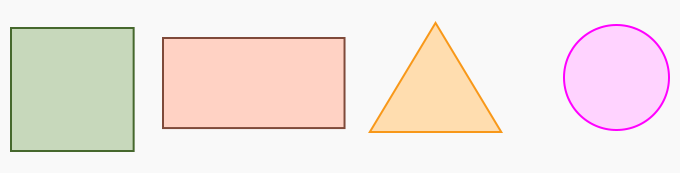The unit of area is called square units. Different shapes have a different formula for calculating the area.

Area of a square and a rectangle:

The area of a square and a rectangle is the product of its two adjacent sides.

 2D Shape Area Formula Example Square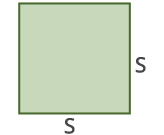Area of a Square = Side × Side Area = S × S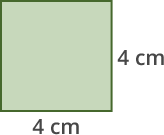Area = 4 × 4 = 16 sq. cm Rectangle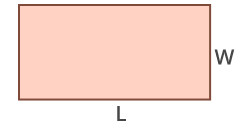Area of a Rectangle = Length × Width = l × w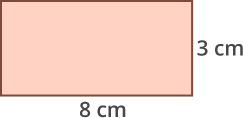Area = 8 × 3 = 24 sq. cm

Area of a triangle:

Triangles could be of various types, like the equilateral triangle, isosceles triangle, and right-angled triangle but the formula for the area of all kinds of triangles is the same.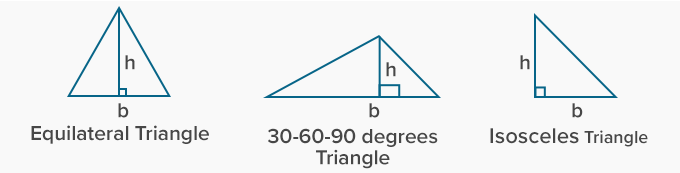We find the area of a triangle using the formula 1× b × h, where base (b) is the length of any one side of the triangle and height (h) is the perpendicular distance between the base and the top vertex of the triangle.

Example:In the triangle ABC, the base measures 6 units and the height measures 4 units.

So, the area of triangle ABC =  12 × b × h

= 12 × 6 × 4

=12 sq units

Circle:

We calculate the area of a circle by the formula π × r2  , where r is the radius of the circle and π is a constant whose value is 227 or 3.14Example: Area of the above circle = π × r2

= 3.14 × 42

= 3.14 × 16

= 50.24 sq. cm

Rhombus:The formula to find the area of a rhombus is pq/2 where p and q are the two diagonals of the rhombus.

In the rhombus ABCD, we can calculate the area as follows:

Area of rhombus = 1pq

= 1× 3 × 5

= 7.5 sq cm

Parallelogram:

To find the area of a parallelogram, we use the formula b × h, where b stands for base and h represnts height. The height is the vertical distance between the base and the top.

Example:In the above figure, the area of the parallelogram is b × h. Therefore it is 6 × 4 = 24 sq. cm

 Fun Facts Among all the shapes with the same perimeter, a circle has the largest area.

Won Numerous Awards & Honors